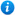* Watermarks do not appear on purchased resources

Preview resource: Click slides to enlarge

# Introducing Prime Numbers - Year 5

Introducing Prime Numbers - Year 5: KS2 Maths Teaching Resources

Year 5 maths programme of study (Number - multiplication and division):

• know and use the vocabulary of prime numbers, prime factors and composite (non-prime) numbers
• establish whether a number up to 100 is prime and recall prime numbers up to 19

In this teaching resource, pupils are introduced to prime numbers as per the curriculum objectives listed above. Content includes:

• What are prime numbers explanation
• What are composite numbers explanation
• Prime number facts
• Using counters to find the factors of given numbers and finding what they have in common
• A how to spot prime numbers explanation
• A sorting numbers activity and accompanying worksheet
• Finding prime numbers activity and worksheet
• A prime and composite number timed activity
• Further prime number worksheet
'Introducing Prime Numbers - Year 5' can be edited so teachers are able to adapt the resource to suit all abilities.

'Introducing Prime Numbers - Year 5' can be previewed in full by clicking on the images above.

Get access to thousands of pages of resources. Find out more about membership here.

Our Price : £2.99 / 3 CreditsBy continuing to use the site, you agree to the use of cookies. You can change this and find out more by following this link